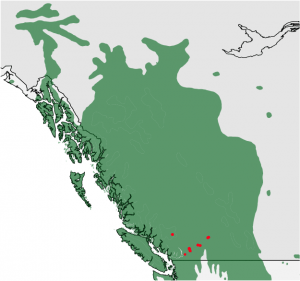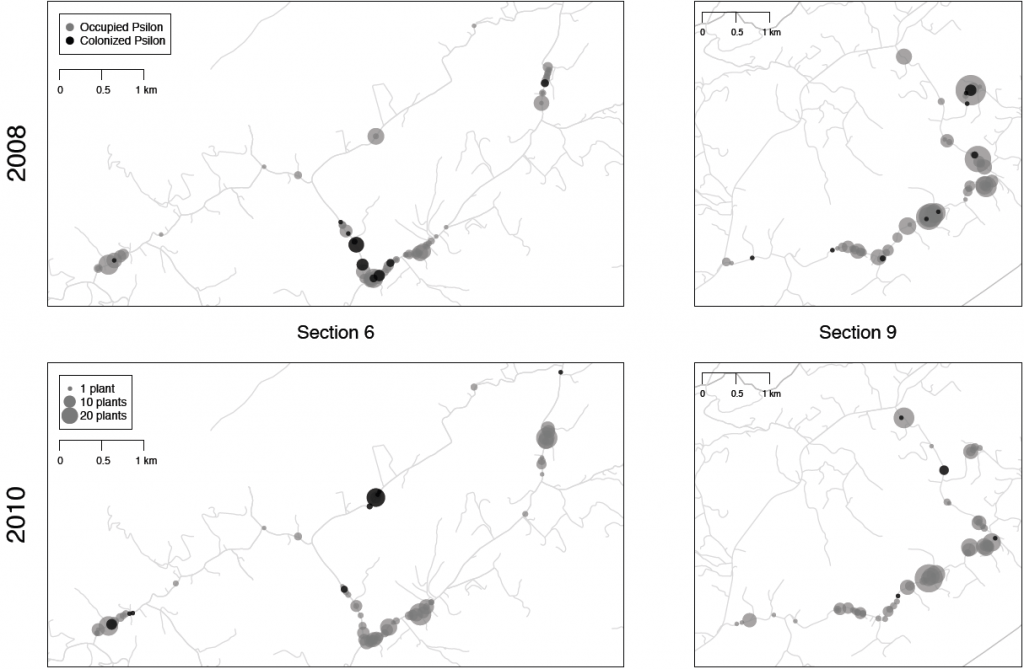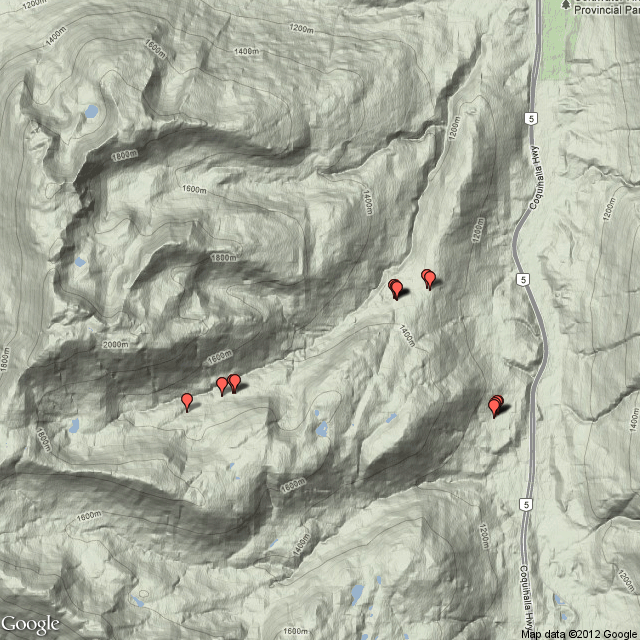# Making Maps with R

First off, thanks to Tim and Jeremy for the invitation to write a guest post here on using R to make maps! As a brief introduction, my name is Kim Gilbert, and I am a Ph.D. student at the University of British Columbia working with Mike Whitlock. I am broadly interested in population genetics and population structure, and am currently studying local adaptation in a tree species. If you want to know more, check out my website, where I also have this tutorial as a .pdf presentation.

Okay, onward with R! And apologies in advance that the R code provided will not show color coded text (a limitation of wordpress), but I decided that it is more useful to leave as text in order to allow copying and pasting rather than insert screenshots that might look nicer but require retyping on your part.

In the field of molecular ecology we see many, many maps. Maps are useful visual tools, from displaying sample sites to performing spatial analyses. To begin, you may ask “why would I want to make a map with R?” There are many answers to this question. R is one of several methods you could choose to make a map. The other main option, or at least most well-known, is ArcGIS, a software suite made by ESRI that includes ArcMap. ArcGIS is great; it is super powerful and has a lot of amazing features, but is not free. It requires a license that many universities provide to their students, however even with this license, it is quite a large suite of programs to install on your local computer and can be frustrating to work with through a remote connection.

R is free, it is open source, and users are constantly contributing new packages and functions. There is always talk of the steep learning curve in R, but I think the ArcGIS learning curve is just as steep if not more so. After taking a semester-long course that only taught ArcMap, I still barely grazed the surface of the capabilities of the software. And because I am not making maps every day, I have quickly forgotten many of the functions within ArcMap. With R, I can write a well-annotated script and then come back to it months or years later and say “ah, yes, that’s how I did this, let me do that now with my new data.” If you are already an R user, then making maps will be quite simple and intuitive since many of the functions take the same or similar arguments as plotting other types of figures. Plus, you have all of R’s online help which is an incredible wealth of knowledge and is how I’ve learned everything I’m about to tell you. And not to harp on it, but one last favorite reason for making maps in R is that it is a cinch to go back to my data file and add or edit a sample point, re-run my script, and bam: I have a new map that is identical to my old one plus my newly added or edited point. I’m done in seconds.

Make a Simple Map

Now that I have successfully convinced you that you want to use R to make your next map, I will show you how. To start, let’s just make a blank map with none of our own data. For this you need two packages: ‘maps’ which contains the functions we will use and ‘mapdata’ which has some basic world map data. Then with one line of code, you can create a simple map, in this case, of Canada:```library(maps)
library(mapdata)
map("worldHires","Canada”, xlim=c(-141,-53), ylim=c(40,85), col="gray90”, fill=TRUE)```

The function ‘`map()`‘ is what is doing the work here. We tell it to plot a map of “Canada”, out of the database “worldHires”. Just like for any other plot in R, we can specify the range of what we want to see, where the extent of our map is in terms of latitude and longitude. It is important to note here that the x-axis is longitude and the y-axis is latitude, which may be counter-intuitive to some since we think in terms of x then y, but we talk of GPS coordinates in terms of lat. then long. Then we can specify a color if desired, and in this case I chose to fill the map with a shade of gray.

All right, so that was easy enough. You can play around with the extent of that map by changing the xlim and ylim, which will allow you to zoom in on parts of Canada. Also check out the maps and mapdata documentations to see what data is already available for mapping other regions.

Plotting GPS Data & Shapefiles

Now to add some of our own data to a map. A lot of the time maps in presentations simply plot sample sites which is very simple to do. All you need is a .csv file of all of your points consisting of at the bare minimum two columns: one for latitude, and one for longitude. Feel free to use my same FieldSamples.csv file to produce the map below. GPS points must be converted into decimal degrees. R will not read points in degrees, minutes, and seconds. For this example, I will add one other layer of data in addition to some of my own sample sites to show the species range.

My data for the species range is contained in what is called a shapefile. If you have never made a map before, I will quickly explain. A shapefile contains a layer of data that can be in the form of polygons, lines, or points, e.g. land zones, roads, or cities, respectively.  (If you work with trees, the USGS has a great source of species ranges here; just watch for when they’ve last been updated.) A lot of GIS data is freely available online with a bit of searching. To read in data from a shapefile, we will make use of the ‘maptools‘ package, and depending on what type of data is contained within your shapefile will determine how it is read in. Polygon data uses ‘`readShapePoly()`‘, line data uses ‘`readShapeLines()`‘, and point data uses ‘`readShapePoints()`‘. After reading in a shapefile, it is added to my map by using the ‘`plot()`‘ function. Then for the GPS data, I read in my .csv file as I would for any other purpose, and I use the ‘`points()`‘ function to plot the points based on my two columns of longitude and latitude.  I also load in a fourth package (‘scales‘) that I will explain below.

```library(maps)
library(mapdata)
library(maptools)  #for shapefiles
library(scales)  #for transparency
pcontorta <- readShapePoly("pinucont.shp")   #layer of data for species range
samps <- read.csv("FieldSamples.csv")   #my data for sampling sites, contains a column of "lat" and a column of "lon" with GPS points in decimal degrees
map("worldHires","Canada", xlim=c(-140,-110),ylim=c(48,64), col="gray90", fill=TRUE)  #plot the region of Canada I want
map("worldHires","usa", xlim=c(-140,-110),ylim=c(48,64), col="gray95", fill=TRUE, add=TRUE)  #add the adjacent parts of the US; can't forget my homeland
plot(pcontorta, add=TRUE, xlim=c(-140,-110),ylim=c(48,64), col=alpha("darkgreen", 0.6), border=FALSE)  #plot the species range
points(samps\$lon, samps\$lat, pch=19, col="red", cex=0.5)  #plot my sample sites```

Details to note from this process: whencreating the map, everything goes in order. I plotted the parts of Canada and the U.S. I wanted first, and this creates the full extent of my final map. I then plotted my species range on top of this map. This is where the ‘scales’ library came in handy, as it provides an easy way to make transparent colors. I wanted to preserve the coastlines and borders behind my species range, so using the function ‘`alpha("darkgreen", 0.6)`‘ tells R that I want this color to be 40% transparent (60% opaque). This could also easily be accomplished without transparency by plotting the countries again on top but with no fill. You can see that for all steps after the first, I have included ‘`add=TRUE`‘ because data are being added to the existing plot, and otherwise there would be no map upon which we are plotting the data. This is important to note because data are being plotted in layers, so things can be covered up by something that is plotted later down the line. Then lastly I plotted my GPS points. You can add some final touches such as drawing a box around the map with ‘`box()`‘, adding text with ‘`text()`‘ and specifying a GPS point as the x and y coordinates of where to place the text, or adding a scale bar with ‘`map.scale()`‘ which brings me to our next important topic.

Projected Maps

When plotting a map such as the one above where I am showing quite a large area, and I am also looking at a more northerly region of the globe, there is distortion that occurs. As we all learned way back when, a globe is the most accurate representation of the Earth’s surface. When we can’t look at a globe, we can use map projections. On my unprojected map above, if I plot a scale bar, depending upon what spot on the map I choose for its location will determine what it conveys.  If I plot it at the top, it makes Vancouver Island (the island at the bottom left of the map) appear to be much smaller than it actually is, and if I plot the scale at the bottom of the map, it makes the top of my map look like it is covering a much larger area than it actually is. Many thanks to J.S. Moore for pointing this out to me and helping to figure out plotting points on projected maps. There is a catch here, in that to the best of my knowledge it is still not possible to draw a map scale on a projected map (but if anyone does know, please share). Instead, when making a projected map, it is possible to draw grid lines on your map.

The package ‘mapproj‘ allows the creation of projected maps, and it includes many different projections that can be chosen from to best suit your needs. In this example, we use the ‘gilbert’ projection:

```library(mapproj)
map(database= "world", ylim=c(45,90), xlim=c(-160,-50), col="grey80", fill=TRUE, projection="gilbert", orientation= c(90,0,225))
lon <- c(-72, -66, -107, -154)  #fake longitude vector
lat <- c(81.7, 64.6, 68.3, 60)  #fake latitude vector
coord <- mapproject(lon, lat, proj="gilbert", orientation=c(90, 0, 225))  #convert points to projected lat/long
points(coord, pch=20, cex=1.2, col="red")  #plot converted points```

The ‘gilbert’ projection requires anadditional parameter, ‘orientation’, telling R where the North Pole is located, which R needs in order to plot correctly. Extra parameters such as this will vary with the projection you choose. Once we’ve plotted our projected map, we want to add our points to it, but if we try this the way I just showed on the previous map, it will not work. The points must first be converted into the chosen projection, and then can be plotted on the map. This is done in the last two lines of code above, from the two vectors of fake points created in the preceding two lines. The function ‘`mapproject()`‘ takes our vectors of latitude and longitude and with the same projection information converts our points to the new object ‘coord’ which we then plot as we would normally. The function ‘`grid.lines()`‘ can then be used to add grid lines.

More R

That covers the gist of basic map-making in R. There are many other packages for mapping or useful tools in conjunction with creating maps. Graduated symbols and colors can easily be made if you have a column of data associated with your GPS points by using that column within your ‘`cex`‘ or ‘`col`‘ specifications. A map legend is plotted the same as any other plot in R, except plotting location is given in terms of a GPS point on the map. Multiple maps can be arranged, for example to create insets, with the ‘`layout()`‘ function. And yes, you can also plot pie charts on a map! This is done through either the ‘plotrix’ package or the ‘mapplots’ package and using the functions ‘`add.pie()`‘ or ‘`floating.pie()`‘ respectively. And those familiar with R know that all of the images you create can be output easily to a file such as a .pdf by bracketing your code with ‘`pdf()`‘ and ‘`dev.off()`‘.Using the ‘layout()’ function to arrange maps in a .pdf
mat <- matrix(c(1,2,3,4),2, byrow=TRUE)
layout(mat, c(8.9782, 5.45), c(6,6))
par(mar=c(0.05,0.05,0.05,0.05))Plot pie charts on a map using ‘add.pie()’ in the ‘mapplots’ package.
add.pie(z=c(east, west), x=lon, y=lat, radius=sqrt(tot), col=c(alpha(“orange”, 0.6), alpha(“blue”, 0.6)), labels=””)
#z indicates the portions of the pie charts filled by each given type, x & y are coordinates for the point, and radius is to designate the size of the circle for the pie chart
#to plot all points, I run a loop to run through my data one point at a time and make each pie chart; there are most likely more efficient methods

I will introduce one last topic before wrapping this post up because I think this is a useful package. ‘RgoogleMaps’ allows you to plot data points on any sort of map you can imagine seeing (terrain, satellite, hybrid) from using Google Maps in your browser. This can be useful if you want more than simply a blank map with points plotted on it. There are two basic functions: ‘`GetMap`‘ and ‘`GetMap.bbox`‘, where the latter takes care of some of the overhead in the former. With these functions, maps are plotted straight to an output file; they will not show up in your quartz window as before.

```library(RgoogleMaps)
lat <- c(48,64) #define our map's ylim
lon <- c(-140,-110) #define our map's xlim
center = c(mean(lat), mean(lon))  #tell what point to center on
zoom <- 5  #zoom: 1 = furthest out (entire globe), larger numbers = closer in
terrmap <- GetMap(center=center, zoom=zoom, maptype= "terrain", destfile = "terrain.png") #lots of visual options, just like google maps: maptype = c("roadmap", "mobile", "satellite", "terrain", "hybrid", "mapmaker-roadmap", "mapmaker-hybrid")```The above code produces a terrain type map from Google, shown on the right. We can add points onto one of these maps, however it is not as straightforward as the maps made previously. Perhaps it is a limitation of my computer’s memory, but I have also run into errors when attempting to plot more than about 35 data points. But for those who are interested, it would be worthwhile to look into these methods more in depth than I have. To plot points, we create a data frame to contain them in addition to anything that was initially read into R:

```samps\$size <- "small"  #create a column indicating size of marker
samps\$col <- "red"   #create a column indicating color of marker
samps\$char <- ""   #normal Google Maps pinpoints will be drawn
mymarkers <- cbind.data.frame(samps\$lat, samps\$lon, samps\$size, samps\$col, samps\$char)   #create the data frame by binding my data columns of GPS coordinates with the newly created columns
names(mymarkers) <- c("lat", "lon", "size", "col", "char")  #assign column headings
lat <- c(48,60)  #now we are plotting the map
lon <- c(-140,-110)
terrain_close <- GetMap.bbox(lonR= range(lon), latR= range(lat), center= c(49.7, -121.05), destfile= "terrclose.png", markers= mymarkers, zoom=13, maptype="terrain")```There are lots of other R packages and capabilities out there that I have not touched on here, many of which are listed in the .pdf file I mentioned at the start of this post. I hope these examples have been useful, and that many of you will now begin creating your maps in R and exploring all of the other mapping capabilities. Thanks for reading!